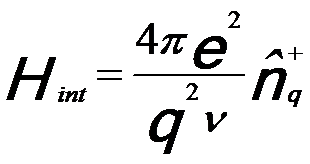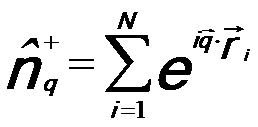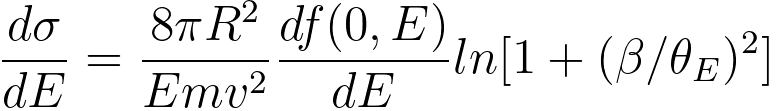Cross-section (Probability) of Inelastic Scattering in EELS Measurements
- Practical Electron Microscopy and Database -
- An Online Book -

http://www.globalsino.com/EM/

=================================================================================

Similar to incident x-rays, energetic incident electrons can also interact with electrons in materials. This process results in an amount ħq of momentum being transferred to the sample. In EELS measurements, the electron interaction is due to coupling between the electrons which may have the form,------------------------------ [3375a]

where,
ν -- A normalization volume for the probe electron ;-- The density fluctuation operator, given by,------------------------------ [3375b]----------------------------------------- [3375c]

Therefore, the double differential scattering cross-section (DDSCS) for inelastic electrons from the ground state |ψi> of the Hamiltonian H0 by applying Fermi's golden rule can be given by,-------- [3375d]

where,
ħω -- The energy lost by the probe electron scattered into solid angle;
m -- The mass of the electron;

Finally, the EEL spectrum can be described in a dielectric formulation  by,-------- [3375e]
where,
v -- The speed of the incident electron.
na -- The number of atoms per unit volume.
θE -- The characteristic scattering angle (θE = E/γm0v2 = E/mv2/2E0).
Im(−1/ε) -- The energy loss function.
a0 -- Bohr radius.
σ -- The total scattering cross section.
Ω -- The solid angle.

Equation 3375e fundamentally presents the probability of a specific scattering event in materials, expressed by the scattering cross-section. This also is the most fundamental equation of EELS. Based on Equation 3375e and a Kramers–Kronig analysis, the complex dielectric function ε = ε1 + iε2 can be obtained from the low-loss EEL spectrum.

For small scattering angles (q → 0), the energy- differential cross-section, up to a collection angle β, can be given by,------------------------ [3375f]
where,
v -- the velocity of the incident electron,
m -- the mass of the incident electron,
R -- the Rydberg energy (see page4433),
E0 -- the incident electron energy.

Equation 3375f is valid within the dipole region, where the GOS (generalised oscillator strength) may be approximated to the optical value f(0,E).

In EELS experimental measurements, the number of atoms in the beam corresponding to, as a function of the core-loss inelastic cross-section, can be given by,-------------------------------------- [3375g]
where,
Δ -- a same energy window above the zero-loss peak and core-loss,
Ic(Δ,β) -- the number of counts into a core-loss edge, i.e. the number of counts above the edge after background is subtracted,
I0(Δ,β) -- the number of counts in the energy window Δ above the zero-loss peak,
σc(Δ,β) -- an appropriate inelastic cross-section obtainable either from theory or from a standard,
β -- the collection semi-angle of EELS,
B -- the effective area of the electron beam.

Table 3375 lists the energy losses and scattering angles of various inelastic electron scatterings in electron interaction with materials.

 Table 3375. Energy losses and scattering angles of various inelastic electron scatterings in electron interaction with materials.
Process Phonon excitation Plasmon excitation (oscillation):
(Introduction)
(Theory)
Direct radiation losses Inner shell ionization
Electrons in outermost shell: only weakly bound to atoms but are coupled to each other by electrostatic forces Intraband transitions Bremsstrahlung radiation due to deceleration of the electron beam in the Coulomb field of an atom Core-loss
Electrons in valence band

Electrons in conduction band

Interband transitions Excitation of loosely bound electrons in conduction band, leading to secondary electrons (SEs) emissions
Thermal diffuse scattering, heating Main transitions of valence electrons 
Special note It normally cannot be resolved in EELS due to the energy spread of the electron source ~20% contribution of peak broadening in EELS at large scattering angles (> 20 mrad)  Most SEs have a kinetic energy of <50 eV; The resulting SE distribution is peaked at 3 to 5 eV, with the distribution decreasing sharply as the energy
increases above 5 eV

Region of energy loss Low loss
(< 50 eV)
Low loss (< 50 eV): Energy loss is low because these electrons require only small amounts of energy for excitation due to loosely bound     High loss (> 50 eV)
Energy loss E [eV] 20 meV–1 eV
3 - 25
10 - 2000
Controlled by the density of loosely bound states
Materials   Predominant in insulators and semiconductors Predominant in metals
EELS background

Predominated by interband transitions of the valence electrons in the absence of
plural plasmon scattering, i.e. in sufficiently thin samples 

EELS broadening   Main origin of peak broadening in EELS 
Interaction   Most common inelastic interaction due to high free electron density;
Inelastic scattering is mainly brought about by the collisions with the loosely bound solid-state electrons

Scattering angle θE [mrad] 5 - 15
< 10
0.1 - 10
Oscillation Collective oscillations of atoms (e.g. lattice vibrations) Collective oscillations of free electrons, a quantum of a collective longitudinal wave in the electron gas of a solid
Detectability by EELS Is not resolved
Yes
Yes
Effects Causes specimen to heat up
Time
Damped out in < 10−15 s

Localization
Localized to < 10 nm

Cross sections
Relatively large
Relatively small
Mean-free
paths

Relatively short
Relatively large
Intensity
Much intense
Much smaller
Modification Can reduce the number of phonons by cooling the specimen

Kikuchi patterns   May be important in the formation of Kikuchi patterns from light elements 
Characteristics Diffuse background, don’t carry any useful information
Signature of the structure
Elemental information

 H. J. Hagemann, W. Gudat, and C. Kunz, Optical Constants from the Far Infrared to the X-ray Region: Mg, Al, Cu, Ag, Au, Bi, C and Al2O3, DESY SR-7417. Desy, Hamburg, W. Germany, 1974.
 W. S. M. Werner Surf. Interface Anal., vol. 31, p. 141, 2001.
 R. F. Egerton, Electron Energy Loss Spectroscopy in the Electron Microscope, Plenum Press, New York, 1996.
 A. P. Stephens, Quantitative microanalysis by electron energy-loss spectroscopy: Two corrections, 5 (1–3) (1980), 343-349.
 R. F. Egerton, Inelastic scattering of 80 keV electrons in amorphous carbon, 31(1), 1975, 199-215.
 Philip, J. G., Whelan, M. J., And Ecerton, R. F.. 1974, Proc. 8th Int. Congress on Electron Microscopy, Canberra. Vol. 1, p. 276.
 P.J. Thomas and P.A. Midgley, An introduction to energy-filtered transmission electron microscopy, Topics in Catalysis, 21 (4), (2002), 109.

=================================================================================